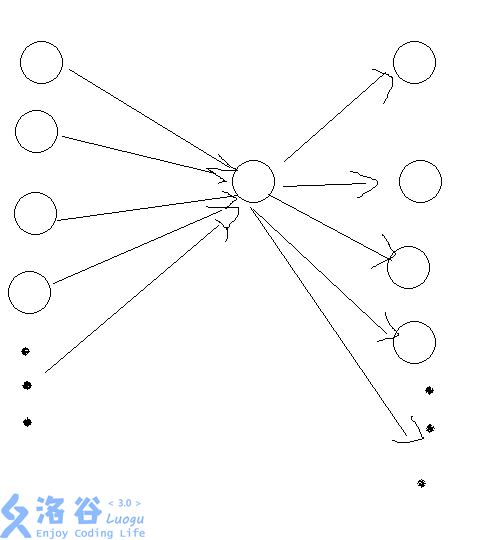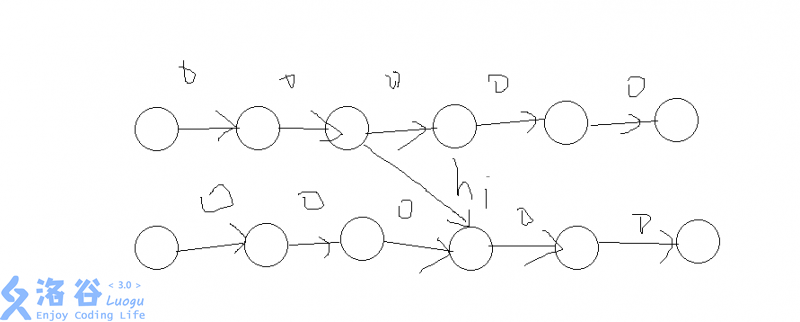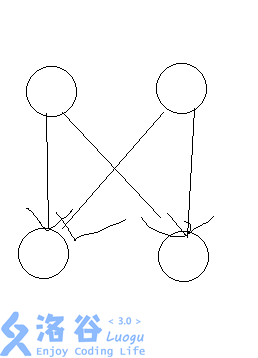### 题解 P3783 【[SDOI2017]天才黑客】

posted on 2018-08-05 15:57:26 | under 题解 |

# 关于spfa

dijkstra保平安

## 本题题解### 前缀和/后缀和优化建图#### 其实是可以的#include<cstdio>
#include<algorithm>
#include<vector>
#include<queue>
using namespace std;
int dfn;int dep;int n;int m;int k;int T;typedef long long ll;
namespace Tree
{
const int N=2*1e4+10;
struct data{int v;int val;friend bool operator <(data a,data b){return a.val<b.val;}};
int fa[N];int df;vector <data> v[N];
inline void add(int u,int V,int w){v[u].push_back((data){V,w});}
inline void dfs(int u)//dfs
{
for(int i=0;fa[u][i];i++)fa[u][i+1]=fa[fa[u][i]][i];
dfn[u]=++df;vector <data> :: iterator it;
for(it=v[u].begin();it!=v[u].end();++it)dep[it->v]=dep[u]+1,fa[it->v]=u,dfs(it->v);
}
inline void pre(){for(int i=1;i<=k;i++)sort(v[i].begin(),v[i].end());dfs(1);}
inline int lca(int u,int v)//倍增lca
{
if(dep[u]<dep[v])swap(u,v);
for(int d=dep[u]-dep[v],i=0;d;d>>=1,i++)if(d&1)u=fa[u][i];if(u==v)return u;
for(int i=17;i>=0;i--)if(fa[u][i]!=fa[v][i])u=fa[u][i],v=fa[v][i];return fa[u];
}
inline void clear()
{
for(int i=1;i<=k;i++)v[i].clear();
for(int i=1;i<=k;i++)for(int j=0;j<18;j++)fa[i][j]=0;df=0;
}
}
namespace Grph
{
const int N=5*1e4+10;const int V=4*N;const int E=20*N;
int tp[V];int ctt;vector <int> eif[N];vector <int> eof[N];vector <int> eib[N];vector <int> eob[N];
vector <int> tr;int v[E];int x[E];int ct;int al[V];int val[E];int s;
inline bool cmp(int a,int b){return dfn[tp[a]]<dfn[tp[b]];}
inline void add(int u,int V,int w){v[++ct]=V;x[ct]=al[u];al[u]=ct;val[ct]=w;}
inline void ins(int u,int V,int w,int d)//插入一个边
{
eof[u].push_back(++ctt);eif[V].push_back(++ctt);eob[u].push_back(++ctt);eib[V].push_back(++ctt);
}
inline void build()
{
for(int u=1;u<=n;u++)
{
sort(eof[u].begin(),eof[u].end(),cmp);sort(eif[u].begin(),eif[u].end(),cmp);
sort(eob[u].begin(),eob[u].end(),cmp);sort(eib[u].begin(),eib[u].end(),cmp);
tr.resize(eof[u].size()+eif[u].size());
merge(eif[u].begin(),eif[u].end(),eof[u].begin(),eof[u].end(),tr.begin(),cmp);
for(int t=0,i=0,j=0;t<(int)tr.size()-1;t++)//然后两两求lca连边
{
if(tr[t]&1)j++;else i++;int w=dep[Tree::lca(tp[tr[t]],tp[tr[t+1]])];
}
}
}
struct data{int u;ll d;friend bool operator <(data a,data b){return a.d>b.d;}};
ll d[V];bool book[V];priority_queue <data> pq;
inline void solve()//跑dijkstra
{
for(int i=1;i<=ctt;i++)d[i]=(1LL<<40);d[s]=0;
for(pq.push((data){s,0});!pq.empty();)
{
data nw=pq.top();pq.pop();if(book[nw.u])continue;book[nw.u]=true;
for(int i=al[nw.u];i;i=x[i])
if(!book[v[i]]&&d[v[i]]>nw.d+val[i])d[v[i]]=nw.d+val[i],pq.push((data){v[i],d[v[i]]});
}
for(int i=2;i<=n;i++)
{
ll ret=(1LL<<40);for(int j=0;j<(int)eif[i].size();j++)ret=min(ret,d[eif[i][j]]);
for(int j=0;j<(int)eib[i].size();j++)ret=min(ret,d[eib[i][j]]);printf("%lld\n",ret);
}
}
inline void clear()
{
for(int i=1;i<=n;i++)eif[i].clear();
for(int i=1;i<=n;i++)eof[i].clear();
for(int i=1;i<=n;i++)eib[i].clear();
for(int i=1;i<=n;i++)eob[i].clear();
for(int i=1;i<=ctt+1;i++)al[i]=0;
for(int i=1;i<=ctt+1;i++)book[i]=false;ct=0;ctt=0;
}
}
inline void solve()
{
scanf("%d%d%d",&n,&m,&k);Grph::s=4*m+1;
for(int i=1,u,v,w,d;i<=m;i++)scanf("%d%d%d%d",&u,&v,&w,&d),Grph::ins(u,v,w,d);
int main(){scanf("%d",&T);for(int z=1;z<=T;z++)solve(),clear();return 0;}//拜拜程序~